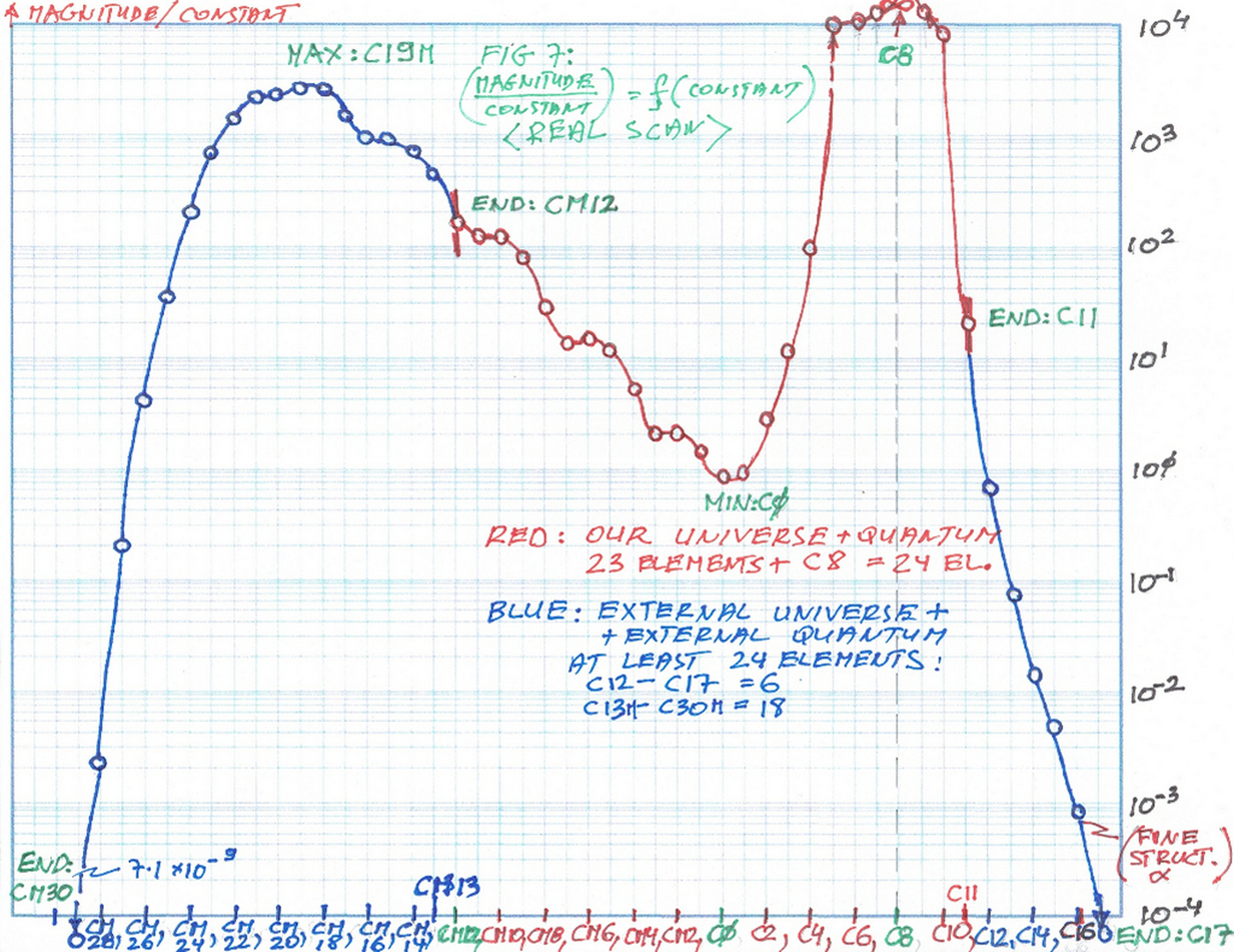7 March 2020 AD; St Thomas Aquinas (1274)

In this article, I will present a graph of the Final Equation of the Construction of the World(s) [contiuous version] and a short description of the graph, as well as a discussion about the implications of the idea of two Universes.

As I stated in the previous article  <  87. The narrative for "The Evidence of another Universe and Quantum..."   >  the main Equation shows clearly another Universe and Quantum consisting of at least 24 elements (the same as our Universe and Quantum). You can see clearly from the graph of the Equation that Our Universe and Quantum (marked in red color) consists of 24 elements plus possibly 2 or 3 coupling constants which may be located outside of our Universe (I'll see into that later). Now, the graph of the Equation continues on both sides - left and right (marked in blue color) and shows clearly another possible System - I claim this is another Universe and Quantum. It consists of at least 24 elements the same as our Universe. The range of Transcendental Constants on the X-axis is from C_Minus_30 (smallest) to C_17 (biggest value of Transcendental Constant). On the Y-axis the results (magnitude of a vector) are divided by the respective Transcendental Constants - for example, the value of the function at C_11 (the end of the range of our Universe and Quantum) will be divided by the value of Transcendental Constant C_11, so the result will be smaller for Constant values greater than 1.0 and bigger for constants less than 1.0.

You can read here about the derivation of the Final Equation of the Construction of the World(s)  part I  Final Equation part I   and here about Final Equation part II  <  Final Equation part II  >

Below is the graph of this Final Equation (just the Real Scan, or the Real part only - it is easier to see the shape of the curve):At the Transcendental Constant C_8 (i.e. π) there is an asymptote (singularity), the value is +infinity. This point, by the way, is one of the three components  (all the elements consists of three pairs) of Time, and it is undefined.

I will not repeat the logical arguments for deducting that part of this curve represents another Universe and Quantum. You can read about it in the article  <  87. The narrative for "The Evidence of another Universe and Quantum..."   >

Statement 1: This is speculation - there are infinitely many universes

Answer: I think there are only two completely different universes - ours and one more beyond (as a Catholic I think it is definitely Heaven). But I have to say very clearly from my math and physics that their structure, elements, and forces will be completely different - you will see it easy on the graph of the function in the next article in a couple of days if you care to read. I am absolutely opposed to a multiverse idea - it is completely wrong. It's just an attempt to get rid of God the Creator.

Statement 2: We have no idea what is out there - our knowledge and technology are not as advanced.

Answer: I agree with technology but I think our minds can grasp it if you use new tools - such as the ones I use - the set of new numbers derived from "pi" and "e". I call them Transcendental Constants. As "pi" let us calculate the length or area etc. of the curved shapes, and "e" gives us the rate of growth or the decay of some processes, all the other Transcendental Constants will give us the ability to calculate precisely some other properties of nature. That is why it is possible to go beyond (with our mind only). These numbers are sort of like the keys to open up some new things, that is why I call them "God's Numbers" or if you prefer "Universe's Numbers" i.e. the numbers the Universe or God for that matter talks to us with. So far we know only two most important these Transcendental Numbers. But you can derive from them ("pi" and "e") whole infinite family. Some of them will rule (i.e. let us calculate exactly) time, space, gravity, etc. Each T. Number has its "specialty" just as "pi" and "e". Using them one can see Universe and Quantum starting "to talk". There is no need for canceling the Quantum Theory as some think we should do, or saying that we cannot figure out our Universe, etc. But this is only the first step - the next one is to derive a Formula (very long equation indeed) which will put all the things together in one place.

You can see how the Transcendental Numbers are derived here  <  15. The derivation of the Transcendental Constants  >

This idea of the dialogue is thanks to my FaceBook friend (I have to give the credit to him for that. His name is Mil S.)

The next articles will be about the calculating of CP Violating Phase Angles of all 8 elements and the Sigma parameters, as well as other stuff.

Comments powered by CComment